Open in App
Not now

# Class 10 RD Sharma Solutions – Chapter 14 Coordinate Geometry – Exercise 14.4

• Last Updated : 30 Nov, 2022

### (i) (1, 4), (-1, -1), and (3, -2)

Solution:

Given, vertices of triangle are (1, 4), (-1, -1), and (3, -2)

As we know that the coordinates of the centroid of a triangle whose vertices are (x1, y1), (x2, y2), (x3, y3)So, the coordinates of the centroid of a triangle are= (1, 1/3)

Hence, the centroid of the triangle whose vertices are (1, 4), (-1, -1), and (3, -2) is (1, 1/3).

### (i) (-2, 3), (2, -1), and (4, 0)

Solution:

Given, vertices of triangle are (-2, 3), (2, -1), and (4, 0)

As we know that the coordinates of the centroid of a triangle whose vertices are (x1, y1), (x2, y2), (x3, y3)So, the coordinates of the centroid of a triangle are= (4/3, 2/3)

Hence, the centroid of the triangle whose vertices are (-2, 3), (2, -1), and (4, 0) is (4/3, 2/3).

### Question 2. Two vertices of a triangle are(1, 2), (3, 5), and its centroid is at the origin. Find the coordinates of the third vertex.

Solution:

According to question

Two vertices of a triangle are(1, 2), (3, 5), and its centroid is at the origin

Find: the coordinates of the third vertex

So, let us assume that the coordinates of the third vertex is (x, y),

So, the coordinates of the centroid of a triangle areAs we know that the centroid is at origin. So,

(x + 1 + 3)/3 = 0

x + 4 = 0

x = -4

(y + 2 + 5)/3 = 0

y + 7 = 0

y = -7

Hence, the third coordinate is (-4, -7)

### Question 3. Find the third vertex of a triangle, If two of Its vertices are at (−3, 1) and (0, −2) and the centroid is at the origin.

Solution:

According to the question

ABC is a triangle in which the coordinates of A are (−3, 1), B are (0, −2), and C are (a, b)

and the centroid of the triangle ABC is (0, 0)

so,

(-3 + 0 + a)/3 = 0

a = 3

(1 – 2 + b)/3 = 0

b = 1

Hence, the coordinates of third vertex is (3, 1)

### Question 4. A (3, 2) and B (−2, 1) are two vertices of a triangle ABC whose centroid G has the coordinates (5/3, -1/3). Find the coordinates of the third vertex C of the triangle.

Solution:

According to the question

ABC is a triangle in which the coordinates of A are (3, 2), B are (−2, 1), and C are (a, b)

and the centroid of the triangle ABC is (5/3, -1/3)

so,

(3 – 2 + a)/3 = 5/3

a = 4

(1 + 2 + b)/3 = -1/3

b = -4

Hence, the coordinates of third vertex is (4, -4)

### Question 5. If (−2, 3), (4, −3), and (4, 5) are the mid-points of the sides of a triangle, Find the coordinates of Its centroid.

Solution: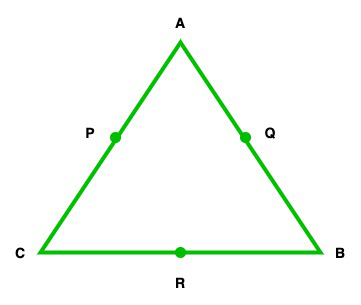Let us assume ABC is a triangle

Now in this triangle P, Q, R be the mid point of side, AC, AB, BC.

The coordinates of P(−2, 3), Q (4, −3), and R(4, 5)

Let us assume that the coordinates of A, B, and C are (x1, y1), (x2, y2), and (x3, y3)

As we know that P is the mid point of side AC,

So,

(x1 + x3) /2 = -2

= x1 + x3 = -4 …..(1)

(y1 + y3) /2 = 3

= y1 + y3 = 6 …..(2)

As we know that Q is the mid point of side AB,

So,

(x1 + x2) /2 = 4

= x1 + x2 = 8 …..(3)

(y1 + y2) /2 = -3

= y1 + y2 = -6 …..(4)

As we know that R is the mid point of side BC,

So,

(x2 + x3) /2 = 4

= x2 + x3 = 8 …..(5)

(y2 + y3) /2 = 5

= y2 + y3 = 10 …..(6)

2(x1 + x2 + x3) = -4 + 8 + 8 = 12  …..( 7)

x1 + x2 + x3 = 6

Now subtract eq(1), (3), (5) from eq(7), we get

x1 = 10

x2 = -2

x3 = -2

Similarly, add (2), (4), and (6), we get

2(y1 + y2 + y3) = -6 + 6 + 10 = 10

y1 + y2 + y3 = 5   ……(8)

Now subtract eq(2), (4), (6) from eq(8), we get

y1 = -1

y2 = 11

y3 = -5

so, the coordinate of  ABC triangle are (10, -1), (-2, 11), and (-2, -5)

Hence, the centroid of the triangle is ((10 – 2 – 2)/3, (-1 + 11 – 5)/3)  = (2, 5/3)

### Question 6. Prove analytically that the middle points of two sides of a triangle is equal to half to the third side.

Solution: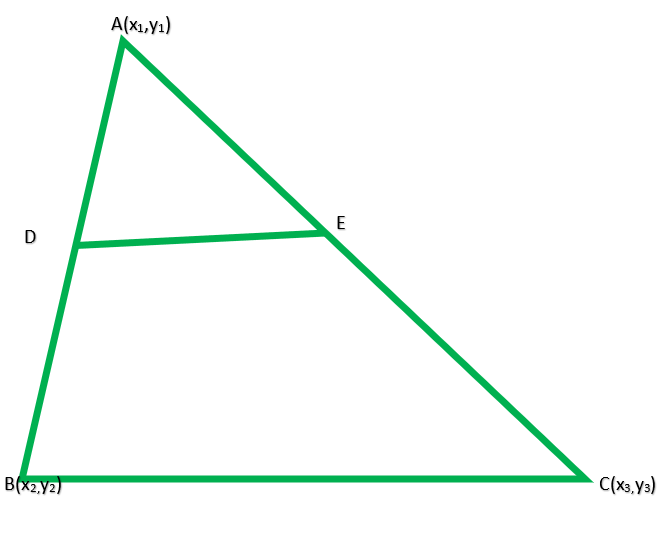In triangle ABC,

D and E are the mid-points of the sides AB and AC

The DE = 1/2 BC

Let the coordinates of the vertices of a ∆ABC be (x1, y2), B(x2, y2), and C(x3, y3)

Then coordinates of D will beand coordinates of E will beLength of BC=……(1)

and the length of DE= 1/2 BC

Hence Proved

### Question 7. Prove that the lines joining the middle points of the opposite sides of a quadrilateral and the join of the points of its diagonals meet in a point and bisect another.

Solution: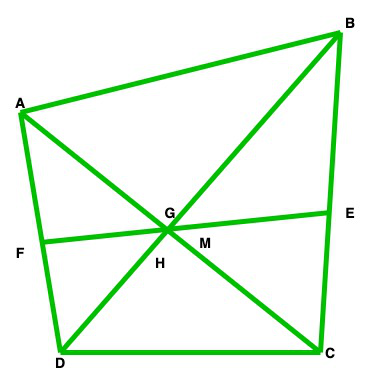Let us assume the vertices of ABCD quadrilateral are A (x1, y1), B (x2, y2), C (x3, y3) and D (x4, y4

In this quadrilateral, E and F are the mid-points of side BC and AD

and EF is joined G and H are the mid-points of diagonal AC and BD.

GH are joined

Now co-ordinates of E will beand co-ordinates of F will beCo-ordinates of G will beCo-ordinates of H will beHere, EF and GH intersect each other at M.

So, let M is the midpoint of EF, then its coordinates will beLet M is the midpoint of GH, then its coordinates of M will beHere, we conclude that the coordinates of in both cases are same

Therefore, EF and GH bisect each other at M

### Question 8. If G be the centroid of a triangle ABC and P be any other point in the plane, prove that PA2 + PB2 + PC2 = GA2 + GB2 + GC2 + 3GP.

Solution: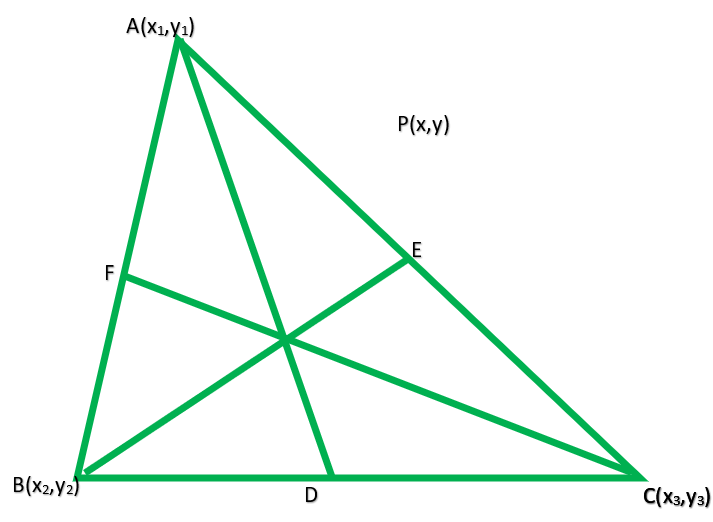According to the question

ABC is a triangle, G is the centroid of it let P(h, x) is any point in the plane.

We have to prove that PA2 + PB2 + PC2 = GA2 + GB2 + GC2 + 3GP

Proof:

In triangle ABC,

Let us assume the coordinates of are(x1, y1) of B are(x2, y2), and of C are(x3, y3)

Therefore, co-ordinates of centroid G will be (u, v)

Where u = (x1 + x2 + 3)/3 and v = (y1 + y2 + 3)/3

Now, we shall find L.H.S and R.H.S. separately

PA2 + PB2 + PC2 = (h – x1)2 + (k – y1)2 + (h – x2)2 + (k – y2)2 + (h – x3)2 + (k – y3)2Hence proved

### Question 9. If G be the centroid of triangle ABC, prove that AB2 + BC2 + CA2 = 3(GA2 + GB2 + GC2).

Solution: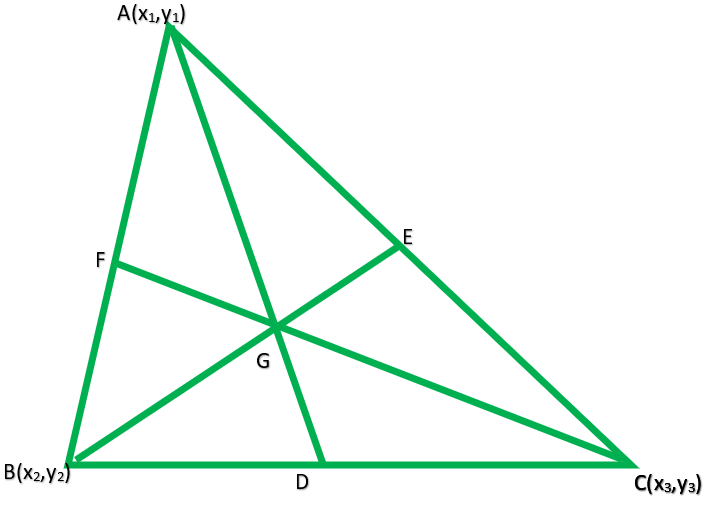According to the question

ABC is a triangle and G be the centroid of this triangle

We have to prove that AB2 + BC2 + CA2 = 3(GA2 + GB2 + GC2)

Proof:

Let us assume that the coordinates of the vertices of ∆ABC be

A(x1, y1), B(x2, y2), and C(x3, y3) and let G be the centroid of the triangle

Therefore, the coordinates of G will beNow L.H.S.= AB2 + BC2 + CA2

= (x1 – x2)2 + (y1 – y2)2 + (x2 – x3)2 + (y2 – y3)2 + (x3 – x1)2 + (y3 – y1)2R.H.S = 3[GA2 + GB2 + GC2]= L.H.S

Hence proved

### Question 10. In the figure, a right triangle BOA is given. C is the mid-point of the hypotenuse AB. Show that it is equidistant from the vertices O, A, and B.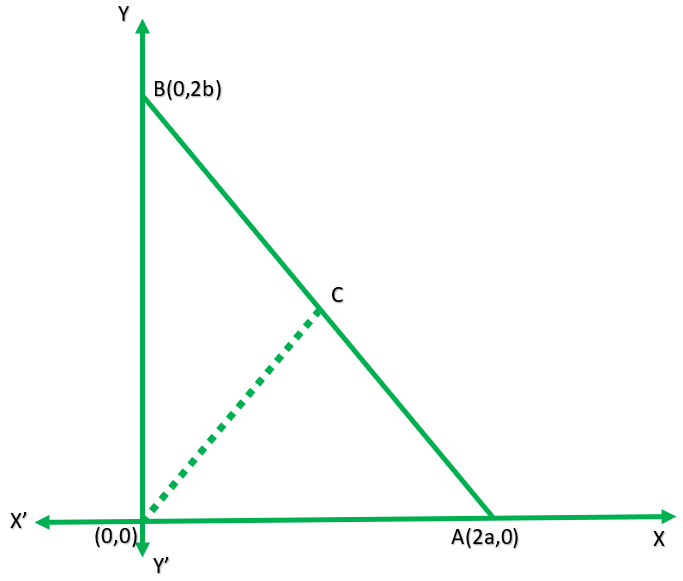Solution:

In right triangle OAB,

Co-ordinates of O are (0, 0), A are(2a, 0), and of B are(0, 2b)

C is the midpoint of AB. So, the co-ordinates of C will beorNow CO =CA =CB =So, we can conclude that, CO = CA = CB

Hence, C is equidistant to form the vertices O, A and B.

Hence proved

My Personal Notes arrow_drop_up